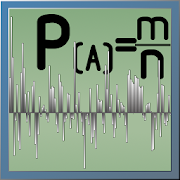Probability theory helps you practice Grade HS Math - Lumos Educational App StorePrice -fREE

DESCRIPTION:

If you toss a coin 5 times, how many times will fall tails? If you roll the dice 7 times, how many times will drop 2? And if you throw a coin and dice to 1 million times? Spend 10 million tests a few seconds on your phone/tablet! The calculator allows you to calculate: - Probability of the event (number of favorable outcomes of all possible outcomes); - Conditional probability of an event; - The relative frequency of the event (number of occurrence of the event, the total number of trials); - Combinatorics: permutations (10 000 members); - Combinatorics: permutations with repetition

OVERVIEW:

Probability theory is a free educational mobile app By .It helps students in grades HS practice the following standards HSS.IC.A.2.

This page not only allows students and teachers download Probability theory but also find engaging Sample Questions, Videos, Pins, Worksheets, Books related to the following topics.

1. HSS.IC.A.2 : Decide if a specified model is consistent with results from a given data-generating process, e.g., using simulation. For example, a model says a spinning coin falls heads up with probability 0.5. Would a result of 5 tails in a row cause you to question the model?.

HS

STANDARDS:

HSS.IC.A.2

Software Version: 1.12

Category:

RELATED APPS

Rate this App?
0

0 Ratings & 0 Reviews

5
0
0
4
0
0
3
0
0
2
0
0
1
0
0EdSearch WebSearch